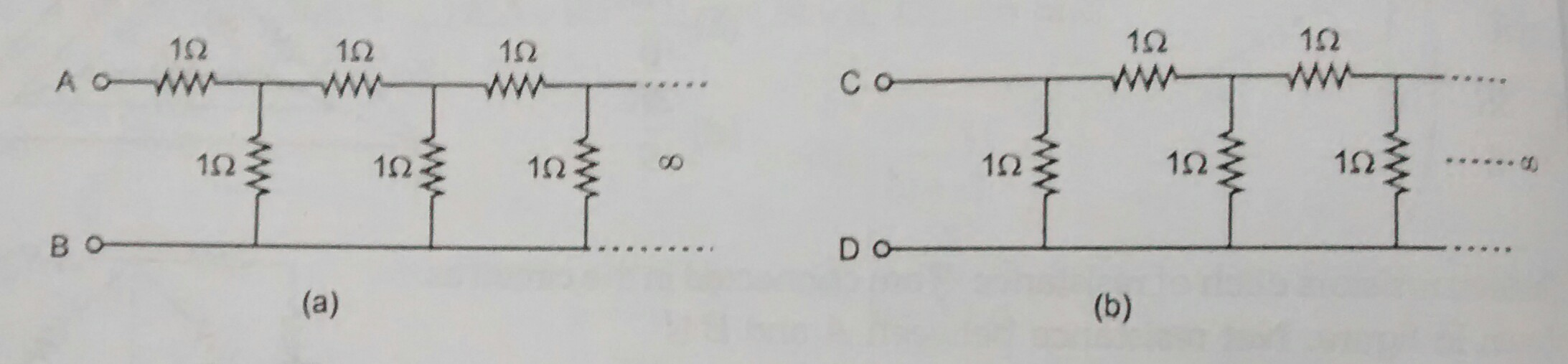# Resistors tend to infinity

According to the 2 circuits shown in the figure which of the following statement is correct:

statement 1: $R_{AB}=R_{CD}=\sqrt{3}+2$

statement 2: $R_{AB}=\sqrt{3}+1$

statement 3: $R_{CD}=\sqrt{5}+1$

sattement 4: $R_{AB}>R_{CD}$Details And Assumptions

• there can be more than 1 correct statements

• If you think that statement statement a and statement b are correct then submit your answer as ab, for example if statement 1 and 2 are correct submit 12, if you think statement 1, 3, 4 are correct submit answer as 134, if only statement 2 is correct submit answer as 2

×